Quantile Regression

Quantile regression generalizes the concept of a univariate quantile to a conditional quantile given one or more covariates. Recall that a student’s score on a test is at thequantile if his or her score is better than that of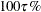of the students who took the test. The score is also said to be at the 100percentile.

For a random variable Y with probability distribution function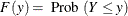thequantile of Y is defined as the inverse function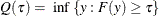where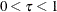. In particular, the median is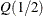.

For a random sample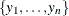of Y, it is well known that the sample median minimizes the sum of absolute deviations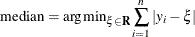Likewise, the generalsample quantile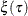, which is the analog of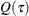, is formulated as the minimizer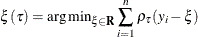where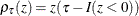,, and where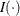denotes the indicator function. The loss function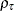assigns a weight ofto positive residuals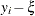and a weight of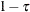to negative residuals.

Using this loss function, the linear conditional quantile function extends thesample quantileto the regression setting in the same way that the linear conditional mean function extends the sample mean. Recall that OLS regression estimates the linear conditional mean function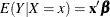by solving for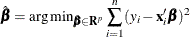The estimated parameter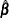minimizes the sum of squared residuals in the same way that the sample mean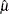minimizes the sum of squares: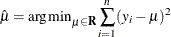Likewise, quantile regression estimates the linear conditional quantile function,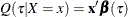, by solving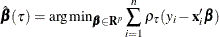for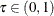. The quantity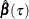is called theregression quantile. The case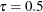, which minimizes the sum of absolute residuals, corresponds to median regression, which is also known as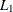regression.

The set of regression quantiles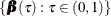is referred to as the quantile process.

The QUANTREG procedure computes the quantile function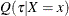and conducts statistical inference on the estimated parameters.